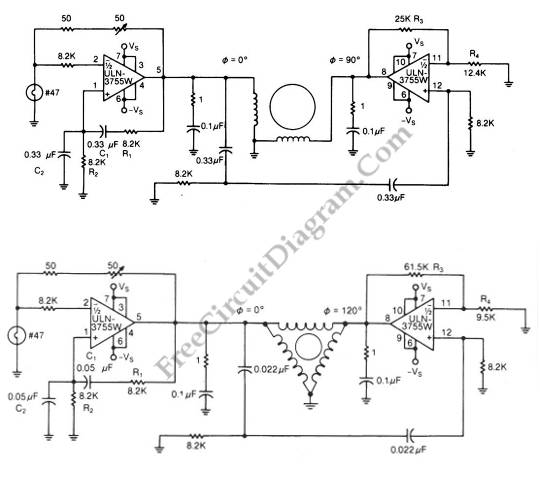# 2 Phase and 3 Phase Motor DriversThe schematic diagram below show 2-phase and 3-phase motor driver circuits. This circuit can used to drive an AC motor because this circuit has an integrated power operational amplifier, built-in power-output stage and high amplification factor. The needed AC signal is produced by the op amp that is configured as oscillator. The motor is driven by the high-current that is supplied from the power output stage. The another op amp is configured as Wien bridge oscillator.  Here are the schematic diagrams of the  circuits:The oscillation frequency is determined by following equation:

f0=1/(2π*squareroot(R1*R2*C1*C2))

The upper schematic diagram is the 2-phase motor driver, while the lower schematic is the diagram of 3-phase motor driver circuit. The oscillator frequency can be adjusted to a narrow range by varying R2 or R1. The signal attenuation  which occurs in the phase shifters is compensated by the second amplifier’s gain. The second amplifier’s gain is set by R3/R4 ratio. The output of this circuit is sinusoidal because of RC feedback networks that used as an active filters. An external source like square wave or pulse can be used to drive this circuit. [Circuit’s schematic diagram source: seekic.com]